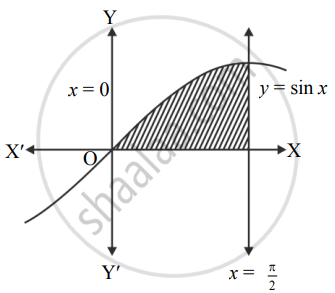# Find the area bounded by the curve y = sin x, the lines x = 0 and x = π2 - Mathematics and Statistics

Sum

Find the area bounded by the curve y = sin x, the lines x = 0 and x = pi/2

#### Solution

Let A be the required area.

Consider the equation y = sin x.∴ A = int_0^(pi/2) y  "d"x

= int_0^(pi/2) sin x  "d"x

= [- cos x]_0^(pi/2)

= -[cos  pi/2 - cos 0]

= – (0 – 1)

∴ A = 1 sq.unit

Concept: Area Bounded by the Curve, Axis and Line
Is there an error in this question or solution?